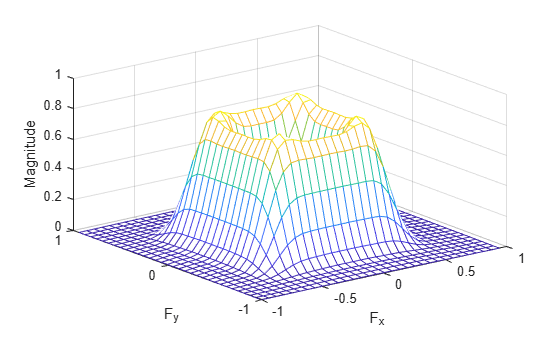# freqz2

2-D frequency response

## Syntax

``[H,f1,f2] = freqz2(h)``
``[H,f1,f2] = freqz2(h,[n1 n2])``
``[H,f1,f2] = freqz2(h,f1, f2)``
``[___] = freqz2(h,___,[dx dy])``
``freqz2(___)``

## Description

example

````[H,f1,f2] = freqz2(h)` returns `H`, the `64`-by-`64` frequency response of `h`, and the frequency vectors `f1` (of length `64`) and `f2` (of length `64`). `h` is a two-dimensional FIR filter, in the form of a computational molecule. `freqz2` returns `f1` and `f2` as normalized frequencies in the range -1.0 to 1.0, where 1.0 corresponds to half the sampling frequency, or π radians.```
````[H,f1,f2] = freqz2(h,[n1 n2])` returns `H`, the `n2`-by-`n1` frequency response of `h`, and the frequency vectors `f1` (of length `n1`) and `f2` (of length `n2`). You can also specify `[n1 n2]` as two separate arguments, `n1,n2`.```
````[H,f1,f2] = freqz2(h,f1, f2)` returns the frequency response for the FIR filter `h` at frequency values in `f1` and `f2`. These frequency values must be in the range -1.0 to 1.0, where 1.0 corresponds to half the sampling frequency, or π radians. You can also specify `[f1 f2]` as two separate arguments, `f1, f2`. ```
````[___] = freqz2(h,___,[dx dy])` uses `[dx dy]` to override the intersample spacing in `h`. You can also specify a scalar to specify the same spacing in both the x and y dimensions.```
````freqz2(___)` produces a mesh plot of the two-dimensional magnitude frequency response when no output arguments are specified.```

## Examples

collapse all

This example shows how to create a two-dimensional filter using `fwind1` and how to view the filter's frequency response using `freqz2`.

Create an ideal frequency response.

```Hd = zeros(16,16); Hd(5:12,5:12) = 1; Hd(7:10,7:10) = 0;```

Create a 1-D window. This example uses a Bartlett window of length 16.

`w = [0:2:16 16:-2:0]/16;`

Create the 16-by-16 filter using `fwind1` and the 1-D window. This filter gives the closest match to the ideal frequency response.

`h = fwind1(Hd,w);`

Display the actual frequency response of the filter.

```colormap(parula(64)) freqz2(h,[32 32]); axis ([-1 1 -1 1 0 1])```## Input Arguments

collapse all

2-D FIR filter, specified in the form of a computational molecule.

Data Types: `single` | `double` | `int8` | `int16` | `int32` | `int64` | `uint8` | `uint16` | `uint32` | `uint64`

Number of points in the frequency response, specified as a two-element vector.

Data Types: `double`

Frequency vectors, specified as numeric vectors.

Data Types: `double`

Sample spacing, specified as a two-element vector of the form ```[dx dy]```. The default spacing is 0.5, which corresponds to a sampling frequency of 2.0. `dx` determines the spacing for the x dimension and `dy` determines the spacing for the y dimension. If you specify a scalar, `freqz2` uses the value to determine the intersample spacing in both dimensions.

Data Types: `double`

## Output Arguments

collapse all

Frequency response, returned as a numeric array.

Frequency vector, returned as a numeric vector.

Data Types: `double`

Frequency vector, returned as a numeric vector.